### Public Perception of Climate Change and the New Climate Dice

This year's analysis is for six 11-year periods 1951-1961, 1962-1972, 1973-1983, 1984-1994, 1995-2005 and 2006-2016, with 1951-1980 base period as usual.

<><><><><><><><><><><><><><><><><><><><><><><><><><>

Figure in Author Summary Published in PrintFigure P1. Frequency of occurence (y-axis) of local temperature anomalies divided by local standard deviation (x-axis) obtained by binning all local results for the indicated region and 11-year period into 0.05 frequency intervals. Area under each curve is unity. Standard deviations are for the 1951-1980 period.

<><><><><><><><><><><><><><><><><><><><><><><><><><>

Figure in GISS Popular Science Page [for NH Land]<><><><><><><><><><><><><><><><><><><><><><><><><><>

Updates of Figures Published in PNAS Plus On-Line

Now we use GHCN v3.3.0 and ERSST v5, but our updates will be mostly forcused over the land and ERSST doesn't matter.Figure 1. Jun-Jul-Aug surface temperature anomalies relative to 1951-1980 mean. Number on upper right is the global mean (average over all area with data).Figure 1 but Dec-Jan-Feb. (Last modified: 2018/09/26)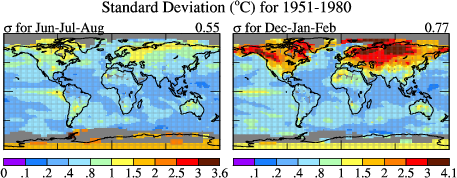Figure 2 Simplified. Standard deviation of local Jun-Jul-Aug (left) and Dec-Jan-Feb (right) mean surface temperature for 30-year-period 1951-1980. (Last modified: 2017/10/03)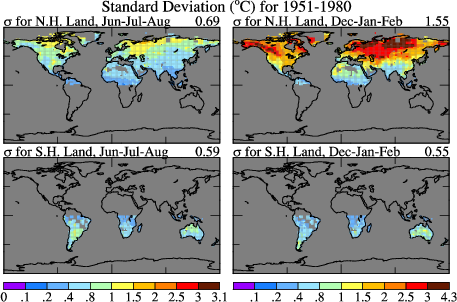Figure 2, but for NH and SH land only. (Last modified: 2017/10/03)Figure 3. Jun-Jul-Aug surface temperature anomalies in units of the local 1951-1980 standard deviation. Numbers above each map are percent of surface area covered by each category in the color bar. (Last modified: 2017/01/23)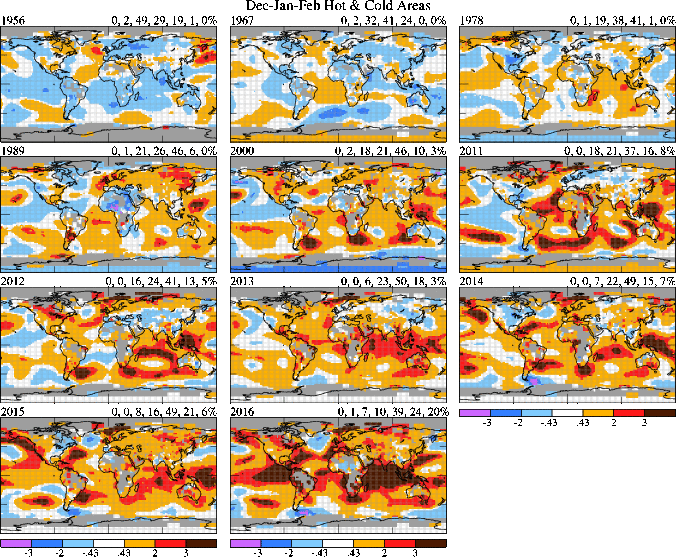Figure 3. but for Dec-Jan-Feb.Figure 6.Figure 6 but Dec-Jan-Feb.Figure 6 but S. Hemisphere.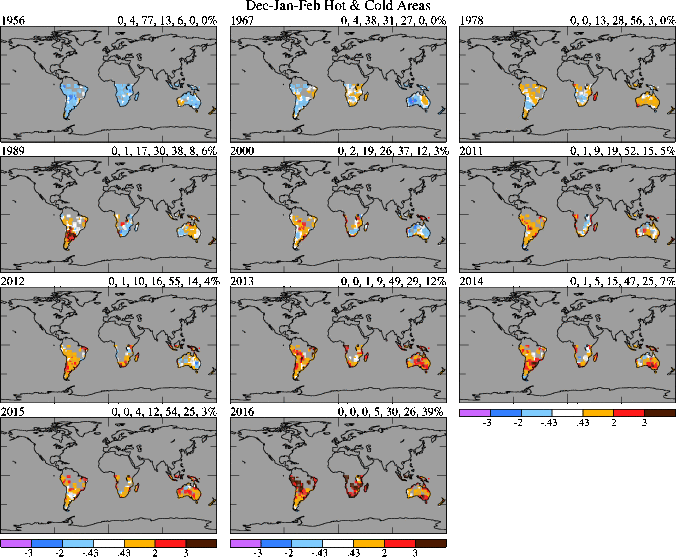Figure 6 but Dec-Jan-Feb in S. Hemisphere.Figure 5. Area covered by temperature anomalies in the categories defined as hot (> 0.43σ), very hot (> 2σ), and extremely hot (> 3σ) with analogous divisions for cold anomalies. Anomalies are relative to 1951-1980 climatology, with σ also from 1951-1980 data.Figure 7. Same as Fig. 5, but for the United States.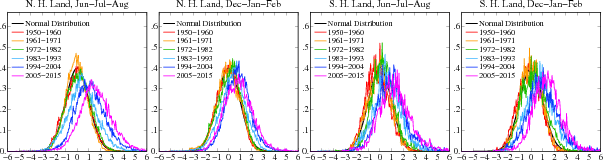Figure 4. Frequency of occurence (y-axis) of local temperature anoma lies (relative to 1951-1980 mean) divided by local standard deviations (x-axis) obtained by counting how many gridboxes have anomalies within each 0.05 interval over 11-year periods. Area under each curve is unity.Figure 8. Jun-Jul-Aug and Dec-Jan-Feb temperature anomalies (°C) for area shown on the right. (Last modified: 2011/10/18)

<><><><><><><><><><><><><><><><><><><><><><><><><><><><><><><><><>
Figures below will not be UpdatedFigure 9. Frequency of occurrence (y-axis) of local temperature anomalies divided by local standard deviation (x-axis) obtained by binning all local results for 11-year periods into 0.05 frequency intervals. Area under each curve is unity. Standard deviations are for the indicated base periods. (Last modified: 2012/01/19)Figure S1. Jun-Jul-Aug surface temperature anomalies in 1931, 1934, 1936, 1941, 1947, 1953. Number on upper right is the global mean (average over all area with data). (Last modified: 2012/03/26)Figure S2. Jun-Jul-Aug surface temperature anomalies over land in 1931, 1934, 1936, 1941, 1947, 1953 relative to 1951-1980 mean temperature in unit of the local 1951-1980 standard deviation of temperature. Numbers above each map are the percent of surface area covered by each category in the color bar. (Last modified: 2012/03/26)